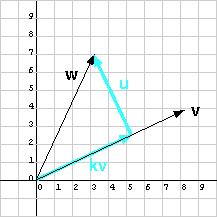Was it actually necessary to compute |w|?

No. It cancelled out of the final result.

# Another ExampleYou don't have to compute the length of w. It cancels out before the final answer. And, all you need is the square of the length of v, |v|2. Some books show formulae for projection that make use of these facts (but, to my taste, are less intuitive).

Here is another example, this time not so easy. The vector w is represented by by (3.2, 7)T. The vector v is represented by by (8, 4)T. Find kv and u.

1. Compute the lengths:
| w |  =   (keep it symbolic)
| v |2  =   (8, 4)T·(8, 4)T   =   80
2. Compute the unit vectors:
wu  =   (3.2, 7)T / | w |
vu  =   (8, 4)T / | v |
3. Compute the cosine of the angle between the vectors:
wu · vu  =   (3.2, 7)T / | w | · (8, 4)T / | v |
=   53.6/( | w || v |)
4. Assemble the projection:
kv  =   | w | (wu·vu) vu
kv  =   | w |   [53.6 / (| w || v |)]   (8, 4)T / | v |
kv  =   53.6 / (| v |)   (8, 4)T / | v |
kv  =   53.6 / (| v |2)   (8, 4)T
kv  =   53.6 / 80   (8, 4)T
kv  =   ((53.6*8)/80, (53.6*4)/80)T  =   ( 5.3, 2.68)T
5. Compute the orthogonal vector:
u  =   w - kv
u  =   (3.2, 7)T - (5.3, 2.68)T  =   (-2.1, 4.32)T

### QUESTION 9:

Does w   =   kv + u, as it should?